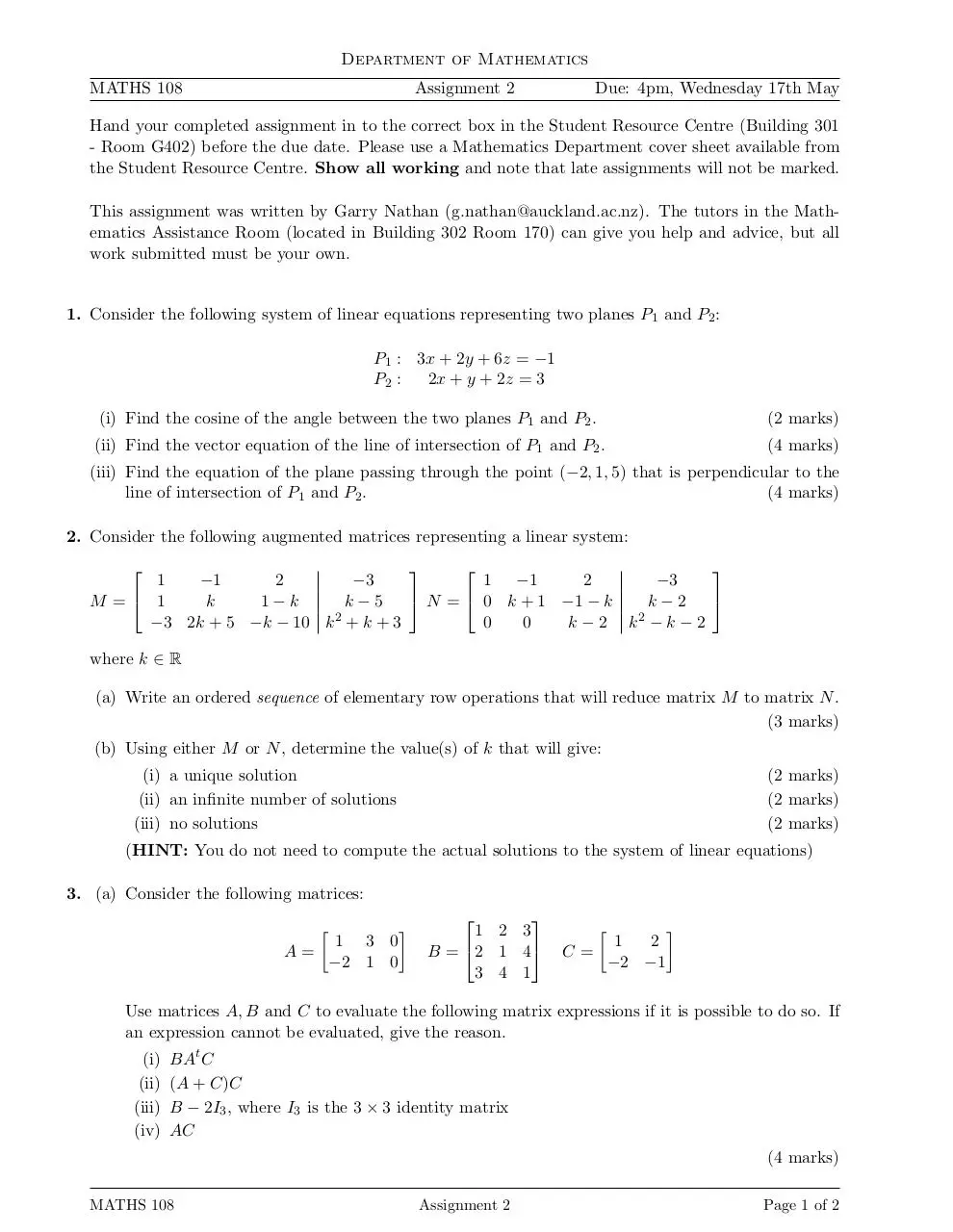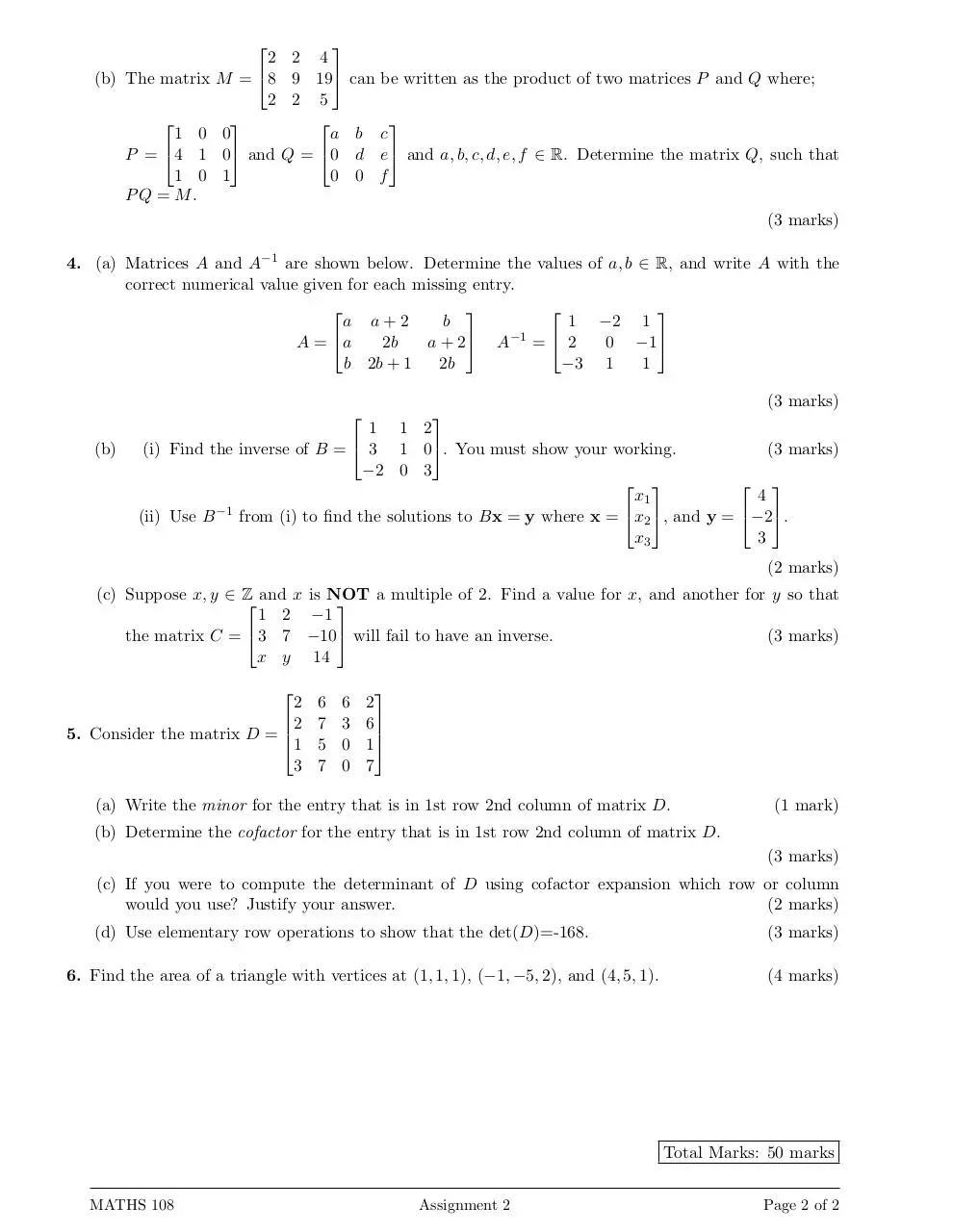# Ass2 2017 S1 .pdf

### File information

This PDF 1.5 document has been generated by TeX / pdfTeX-1.40.14, and has been sent on pdf-archive.com on 11/05/2017 at 01:17, from IP address 202.36.x.x. The current document download page has been viewed 1196 times.
File size: 114.86 KB (2 pages).
Privacy: public file

### Document preview

Department of Mathematics
MATHS 108

Assignment 2

Due: 4pm, Wednesday 17th May

Hand your completed assignment in to the correct box in the Student Resource Centre (Building 301
- Room G402) before the due date. Please use a Mathematics Department cover sheet available from
the Student Resource Centre. Show all working and note that late assignments will not be marked.
This assignment was written by Garry Nathan (g.nathan@auckland.ac.nz). The tutors in the Mathematics Assistance Room (located in Building 302 Room 170) can give you help and advice, but all
work submitted must be your own.

1. Consider the following system of linear equations representing two planes P1 and P2 :
P1 : 3x + 2y + 6z = −1
P2 :
2x + y + 2z = 3
(i) Find the cosine of the angle between the two planes P1 and P2 .

(2 marks)

(ii) Find the vector equation of the line of intersection of P1 and P2 .

(4 marks)

(iii) Find the equation of the plane passing through the point (−2, 1, 5) that is perpendicular to the
line of intersection of P1 and P2 .
(4 marks)
2. Consider the following augmented matrices representing a linear system:

1
−1
2
−3
1 −1
2
−3
 N =  0 k + 1 −1 − k

k
1−k
k−5
k−2
M = 1
2
2
−3 2k + 5 −k − 10 k + k + 3
0
0
k−2 k −k−2
where k ∈ R
(a) Write an ordered sequence of elementary row operations that will reduce matrix M to matrix N .
(3 marks)
(b) Using either M or N , determine the value(s) of k that will give:
(i) a unique solution
(ii) an infinite number of solutions
(iii) no solutions

(2 marks)
(2 marks)
(2 marks)

(HINT: You do not need to compute the actual solutions to the system of linear equations)
3. (a) Consider the following matrices:


1 3 0
A=
−2 1 0



1 2 3
B = 2 1 4
3 4 1




1
2
C=
−2 −1

Use matrices A, B and C to evaluate the following matrix expressions if it is possible to do so. If
an expression cannot be evaluated, give the reason.
(i)
(ii)
(iii)
(iv)

BAt C
(A + C)C
B − 2I3 , where I3 is the 3 × 3 identity matrix
AC
(4 marks)

MATHS 108

Assignment 2

Page 1 of 2

(b) The matrix

1 0
P = 4 1
1 0
PQ = M.

2
M = 8
2

0
0 and
1

2 4
9 19
2 5

a
Q = 0
0

can be written as the product of two matrices P and Q where;

b c
d e  and a, b, c, d, e, f ∈ R. Determine the matrix Q, such that
0 f
(3 marks)

4. (a) Matrices A and A−1 are shown below. Determine the values of a, b ∈ R, and write A with the
correct numerical value given for each missing entry.

a a+2
b
1 −2 1
2b
a + 2 A−1 =  2
0 −1
A = a
b 2b + 1
2b
−3 1
1
(3 marks)

(b)

1 1 2
(i) Find the inverse of B =  3 1 0. You must show your working.
(3 marks)
−2 0 3
 
 
x1
4
(ii) Use B −1 from (i) to find the solutions to Bx = y where x = x2 , and y = −2.
x3
3
(2 marks)

(c) Suppose x, y ∈ Z and x is NOT
a multiple of 2. Find a value for x, and another for y so that

1 2 −1
(3 marks)
the matrix C =  3 7 −10 will fail to have an inverse.
x y 14

2
2
5. Consider the matrix D = 
1
3

6
7
5
7

6
3
0
0

2
6

1
7

(a) Write the minor for the entry that is in 1st row 2nd column of matrix D.

(1 mark)

(b) Determine the cofactor for the entry that is in 1st row 2nd column of matrix D.
(3 marks)
(c) If you were to compute the determinant of D using cofactor expansion which row or column
(2 marks)
(d) Use elementary row operations to show that the det(D)=-168.
6. Find the area of a triangle with vertices at (1, 1, 1), (−1, −5, 2), and (4, 5, 1).

(3 marks)
(4 marks)

Total Marks: 50 marks
MATHS 108

Assignment 2

Page 2 of 2Ass2_2017_S1.pdf (PDF, 114.86 KB)

### Share on social networks

#### HTML Code

Copy the following HTML code to share your document on a Website or Blog